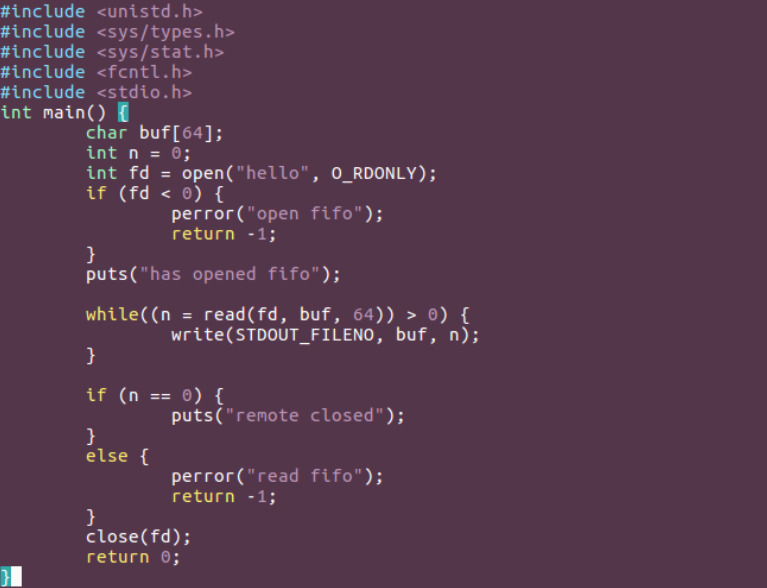# 实验八 进程间通信

1.举例说明使用匿名管道进行进程通信。

man 2 pipe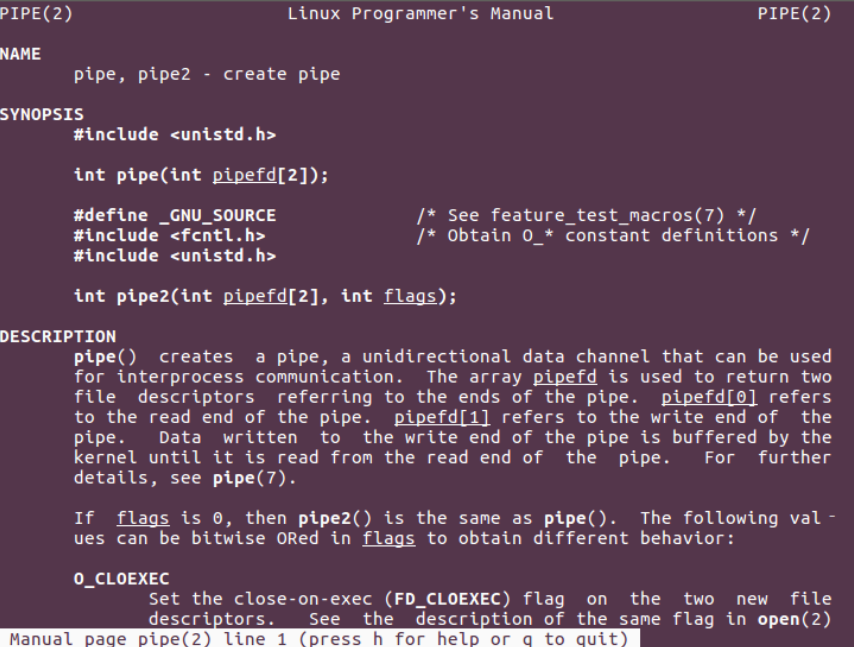pipe 函数打开的文件描述符是通过参数（数组）传递出来的，而返回值表示打开成功（0）或失败 （-1）。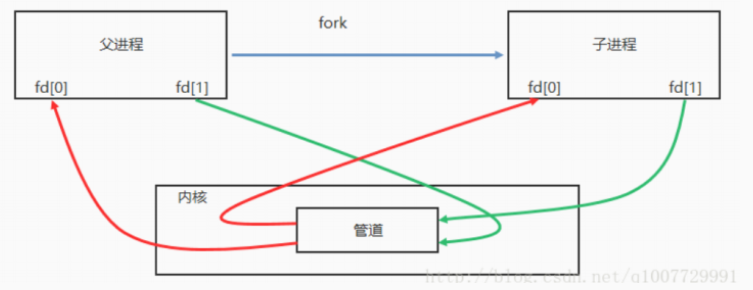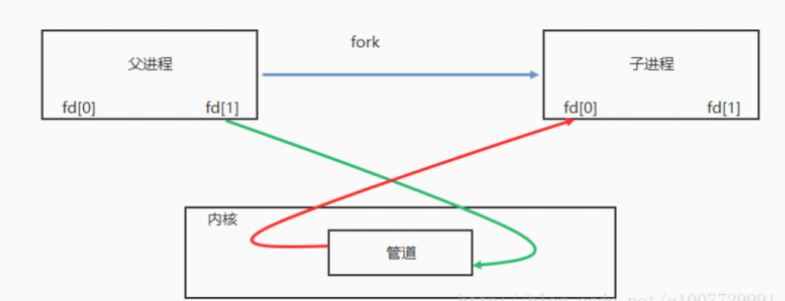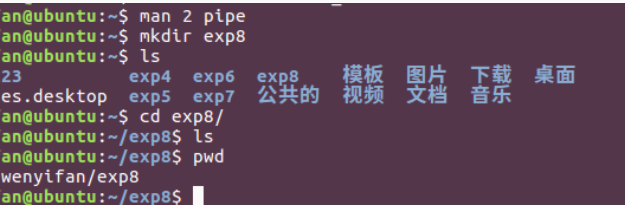//hellopipe.c
#include <stdio.h>
#include <unistd.h>
#include <stdlib.h>
#include <ctype.h>
void child(int *fd) {
close(fd); // 子进程关闭写端
char buf;
int n = 0,i;
while(1) {
for (i = 0; i < n; ++i)
putchar(toupper(buf[i]));
if (*buf == 'q') {
close(fd);
exit(0);
}
if (n == 0) {
sleep(1);
}
}
exit(0);
}
int main() {
int fd;//作为传出参数
int n = 0;
char buf = { 0 };
if (pipe(fd) < 0) {
perror("pipe");
return -1;
}
pid_t pid = fork();
if (pid == 0) {
child(fd);
}
close(fd);// 父进程关闭读端
while (1) {
write(fd, buf, n);
if (*buf == 'q') {
close(fd);
exit(0);
}
}
return 0;
}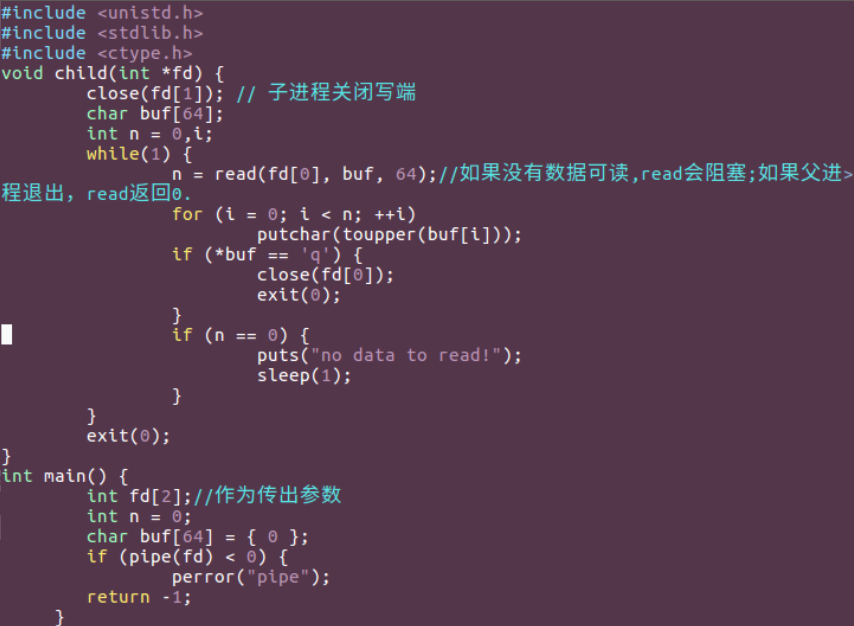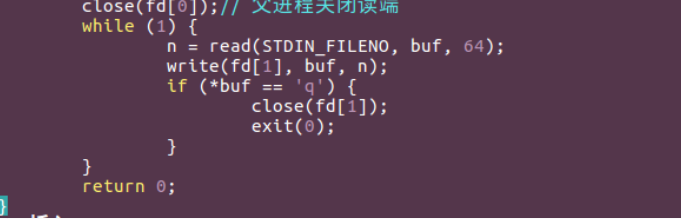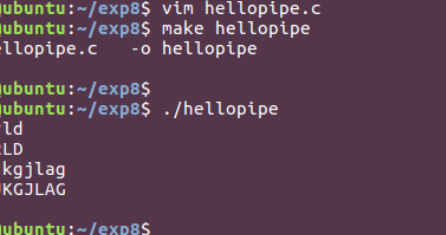2.举例说明使用 mkfifo 命令创建命名管道以及简单演示管道如何工作。

1.通过命令 mkfifo 创建管道

man mkfifo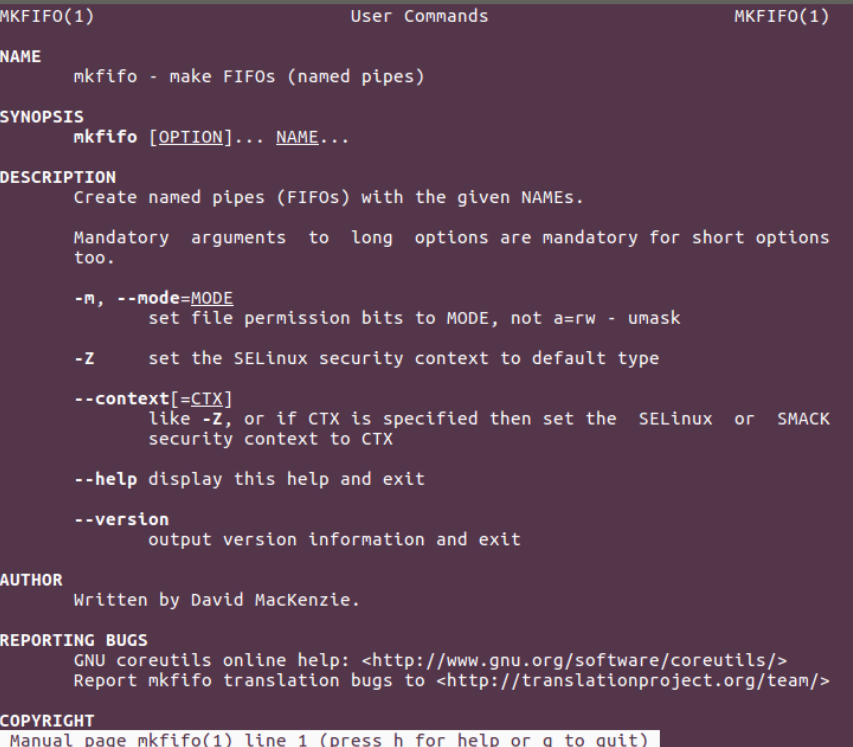2.通过函数 mkfifo(3) 创建管道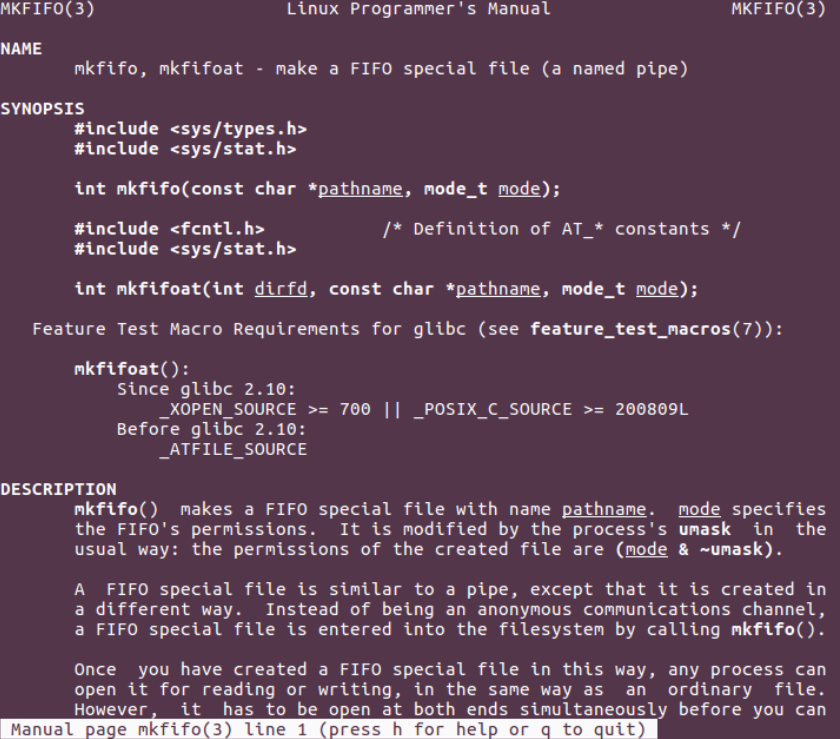FIFO文件的特性
a） 查看文件属性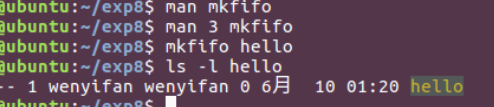b) 使用 cat 命令打印 hello 文件内容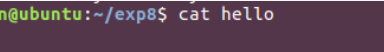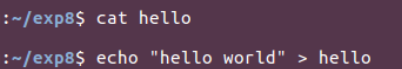c) fifo 文件特性

(1)文件属性前面标注的文件类型是 p ，代表管道
(2)文件大小是 0
(3)fifo 文件需要有读写两端，否则在打开 fifo 文件时会阻塞

3.编写两个程序使用第2题中创建的管道进行通信

// pipe_send.c
#include <unistd.h>
#include <sys/types.h>
#include <sys/stat.h>
#include <fcntl.h>
#include <stdio.h>
int main() {
char buf;
int n = 0;
int fd = open("hello", O_WRONLY);
if (fd < 0) {
perror("open fifo");
return -1;
}
puts("has opend fifo");

while((n = read(STDIN_FILENO, buf, 64)) > 0) {
write(fd, buf, n);
if (buf == 'q')
break;
}
close(fd);
return 0;
}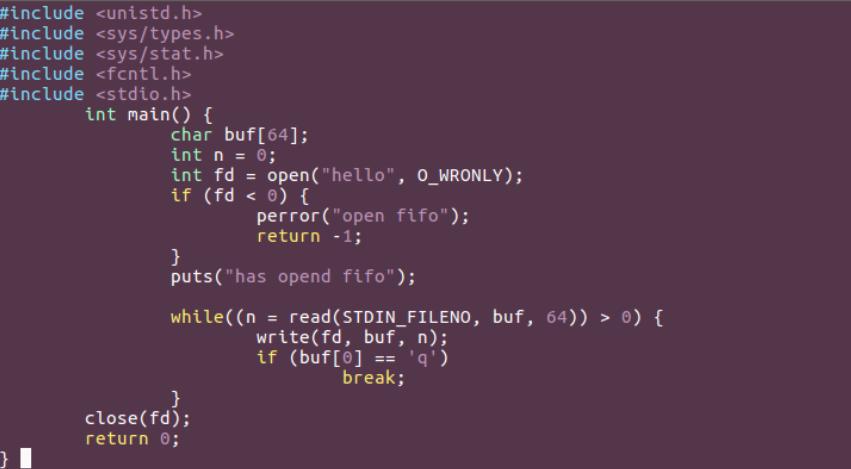// pipe_recv.c
#include <unistd.h>
#include <sys/types.h>
#include <sys/stat.h>
#include <fcntl.h>
#include <stdio.h>
int main() {
char buf;
int n = 0;
int fd = open("hello", O_RDONLY);
if (fd < 0) {
perror("open fifo");
return -1;
}
puts("has opened fifo");

while((n = read(fd, buf, 64)) > 0) {
write(STDOUT_FILENO, buf, n);
}

if (n == 0) {
puts("remote closed");
}
else {